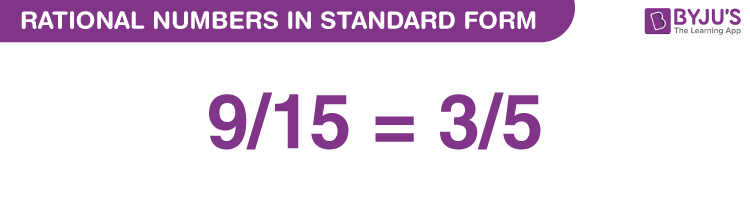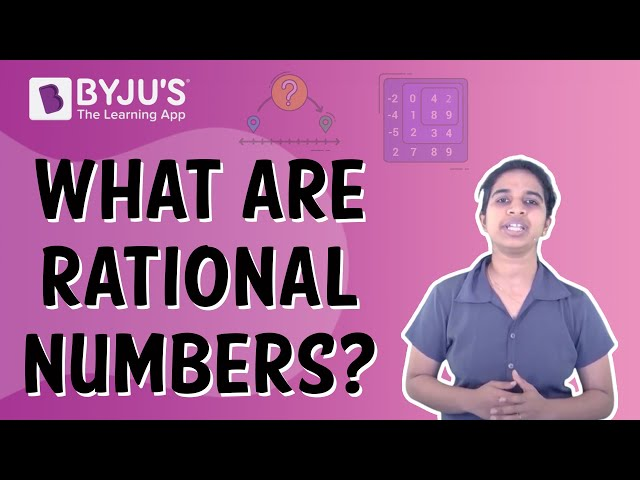# Rational Numbers in Standard Form

Expressing a rational number in its standard form means there is no common factor, other than 1, available in its numerator and denominator and its denominator is a positive integer.  Numbers that can be expressed in the form of p/q, where p and q are integers and q is not equal to zero, are known as rational numbers.  Hence, if 4/6 is a rational number, then its standard form will be 2/3 since we cannot solve 2/3, anymore.In Maths, we rationalize the fractions to express them into standard forms. The fractions have numerator and denominator part. A fraction is in standard form when the numerator and denominator are co-prime. All integers and fractions are rational numbers. It is easy to perform addition and subtraction of rational numbers once we have rationalised their denominators. The standard form of rational number helps us to determine the value in a more specific way. Like, 20/25 can be expressed as 4/5, 10/20 can be expressed as 1/2 and so on.

## How to Identify if a Given Rational Number is in Standard Form?

1. Whenever we have a rational number, first, we find the H.C.F. of numerator and denominator, if it is 1 i.e. if the numerator and denominator of the rational number are coprime numbers, then the given rational number is in its standard form.
2. If the numerator and denominator are not co-prime, then we start dividing both the numerator and denominator by the common factor of both. We keep on dividing the numerator and denominator with the common factors unless we get a numerator and denominator with H.C.F. equal to 1.

## How to Convert Rational Number into Standard Form?

Go through the following steps to convert the given rational number to its standard form.

Step 1: Get the given rational number.

Step 2: Check whether the denominator of the given rational number is positive or negative. If the denominator is negative, multiply or divide both the numerator and denominator by -1, so that the denominator becomes positive.

Step 3: Find the HCF of the absolute value of the numerator and denominator.

Step 4: Divide both numerator and denominator of the given rational number by the HCF value obtained in step 3.

Step 5: The resultant obtained is the standard form of the rational number.

Let us consider the following example, to have a better understanding.

Consider a rational number, 16/24. The H.C.F. of 16 and 24 is 8, which is not equal to 1, hence the given rational number is not in its standard form. Now, we know that 2 is a common factor of 16 and 24.  Dividing both the numerator and denominator by 2, we get 8/12.

Again the H.C.F. is not equal to 1, so again dividing it by 2. On dividing the numerator and denominator by 2 we get 4/6. Still, we find that the H.C.F. is not equal to 1. So, we will again divide both the numerator and denominator by 2. So, now we finally obtain 2/3. The H.C.F. of 2 and 3 is 1, i.e. 2 and 3 are co-prime. Hence, the rational numbers obtained now has an H.C.F. equal to 1.

Therefore, the standard form of 16/24 = 2/3

In this way, we can convert the given rational number into its standard form.

### Rational Numbers to Standard Form Examples

Example 1:

Write 23/69 in standard form.

Solution:

Given, 23/69

If we take out the H.C.F. of 23 and 69, we get;

23 = 1 x 23

69 = 1 x 3 x 23

As we can see here, there is one more factor common between 23 and 69 other than 1, which is 23 itself, therefore if we cancel the common factor from numerator and denominator, we get;

23/69 = 1/3

Example 2:

Which of the following rational numbers is equivalent to 2/3?

(a) 3/2

(b) 4/9

(c) 4/6

(d) 9/4

Solution:

4 = 2 x 2

6 = 2 x 3

Since 2 is the common factor here, then we will cancel it from numerator and denominator.

Therefore, 4/6 = 2/3.

## Video Lesson on Rational NumbersStay tuned with BYJU’S – The Learning App to learn all Maths concepts easily by exploring more videos.

## Frequently Asked Questions on Rational Numbers in Standard Form

Q1

### What is meant by rational numbers in standard form?

A rational number is said to be in standard form if both the numerator and denominator contain no common factors other than 1 and the denominator should have a positive integer.

Q2

### How to convert the rational number to its standard form?

To convert the rational number to its standard form, start dividing both numerator and denominator by the common factor of both. If the HCF of numerator and denominator becomes 1, stop the process.

Q3

### What is the standard form of the rational number 12/18?

The standard form of the rational number 12/18 is 2/3.

Q4

### Why do we use rational numbers in standard form?

Rational numbers in the standard form help us to determine the value in a more specific way such as 3/15 is expressed as 1/5, and so on.

Q5

### What is the standard form of 27/-72?

The standard form the rational number 27/-72 is -3/8.

Test your Knowledge on Rational Numbers in Standard Form